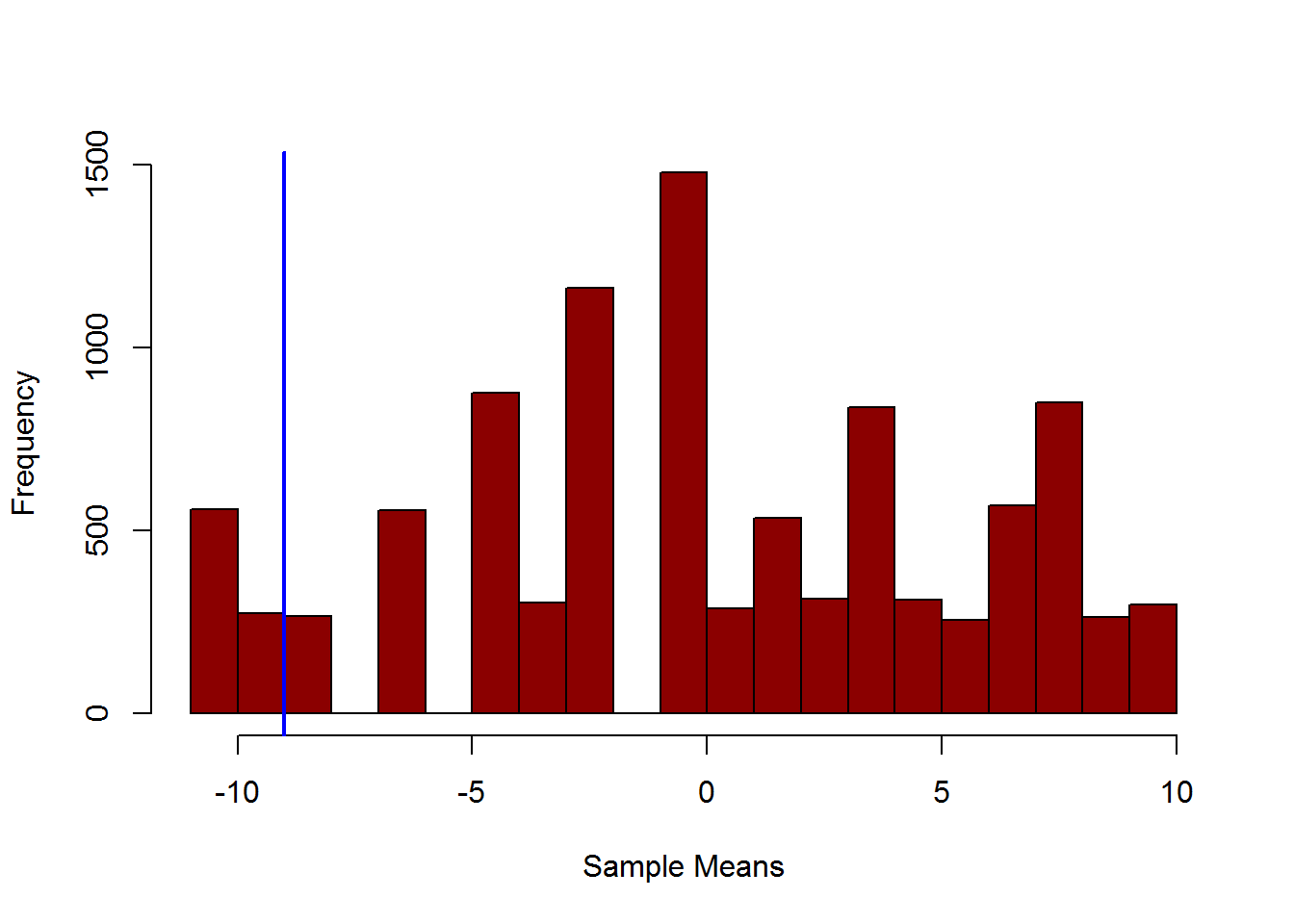The goal is to test whether the observed difference between the treatment and the control samples is really significative.See https://www.youtube.com/watch?v=GmvpsJHGCxQ for more details.

observed.treatment.sample <- c(19,22,25,26) #the observed scores for the treatment group
observed.control.sample <- c(23,33,40) #the observed scores for the control group
observed.mean.difference <- mean(observed.treatment.sample) - mean(observed.control.sample)
observed.mean.difference #the mean difference between the two observed groups
##  -9

Is this difference significative or due to chance?

whole.sample <- c(observed.treatment.sample,observed.control.sample) #put together the two samples
random.mean.differences <- vector() #this vector will be containing the mean differences of each loop iteration
for (i in 1:10000) { #compute 10000 times random differences in the whole sample

random.sample <- sample(whole.sample) #randomized the whole sample
random.treatment.sample <- random.sample[1:4] #selected new random treatment sample
random.control.sample <- random.sample[5:7] #selected new random control sample
#for each iteration of the loop, the new mean difference is added to the vector
random.mean.differences <- c(random.mean.differences,
mean(random.treatment.sample)-
mean(random.control.sample))

}
hist(random.mean.differences, main = "", xlab = "Sample Means", prob = F, col = "darkred")
abline(v = observed.mean.difference, col = "blue", lwd = 2) #the observed value on the histogramCompute how many times our observed difference is equal or greater than a random difference.

p.value.left <- sum(random.mean.differences < observed.mean.difference) / length(random.mean.differences)
p.value.right <- sum(random.mean.differences > observed.mean.difference) / length(random.mean.differences)
p.value.left
##  0.0558
p.value.right
##  0.9168

In the sample studied, the chance of having the observed difference or greater is higher the threshold of 0.025 (left one-tailed testing).